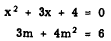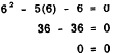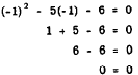Quadratic equations in one variableCustom SearchCHAPTER 16 QUADRATIC EQUATIONS IN ONE VARIABLE The degree of an equation in one variable is the exponent of the highest power to which the variable is raised in that equation. A second degree equation in one variable is one in which the variable is raised to the second power. A second-degree equation is often called a QUADRATIC EQUATION. The word quadratic is derived from the Latin word quadratus, which means "squared." In a quadratic equation the term of highest degree is the squared term. For example, the following are quadratic equations:The terms of degree lower than the second may or may not be present. The possible terms of lower degree than the squared term in a quadratic equation are the first-degree term and the constant term. In the equation         3x2 - 8x - 5= 0 -5 is the coefficient of x0. If we wished to emphasize the powers of x in this equation, we could write the equation in the form         3x2 - 8x1 - 5x0 = 0 Examples of quadratic equations in which either the first-degree term or the constant term is missing are :GENERAL FORM OF A QUADRATIC EQUATION Any quadratic equation can be arranged in the general form:         ax2 + bx + c = 0 If it has more than three terms, some of them will be alike and can be combined, after which the final form will have at most three terms. For example,         2x2 + 3 + 5x - 1 + x2 = 4 - x2 - 2x - 3 reduces to the simpler form         4x2 + 7x + 1 = 0 In this form, it is easy to see that a, the coefficient of x2, is 4; b, the coefficient of x, is 7; and c, the constant term, is 1. Sometimes the coefficients of the terms of a quadratic appear as negative numbers, as follows:         2x2 - 3x - 5 = 0 This equation can be rewritten in such a way that the connecting signs are all positive, as in the general form. This is illustrated as follows:         2x2 + (-3)x + (-5) = 0 In this form, the value of a is seen to be 2, b is -3, and c is -5. An equation of the form         x2 + 2 = 0 has no x term. This can be considered as a case in which a is 1 (coefficient of x2 understood to be l), b is 0, and c is 2. For the purpose of emphasizing the values of a, b, and c with reference to the general form, this equation can be written         x2 + 0x + 2 = 0 The coefficient of x2 can never be 0; if it were 0, the equation would not be a quadratic. If the coefficients of x and x0 are 0, then those terms do not normally appear. To say that the coefficient of x0 is 0 is the same as saying that the constant term is 0 or is missing. A ROOT of an equation in one variable is a value of the variable that satisfied the equation. Every equation in one variable, with constants as coefficients and positive integers as exponents, has as many roots as the exponent of the highest power. In other words, the number of roots is the same as the degree of the equation. A fourth-degree equation has four roots, a cubic (third-degree) equation has three roots, a quadratic equation has two roots, and a linear equation has one root. As an example, 6 and - 1 are roots of the quadratic equation         x2 - 5x - 6 = 0 This can be verified by substituting these values into the equation and noting that an identity results. in each case. Substituting x = 6 givesSubstituting x = -1 givesSeveral methods of finding the roots of quadratic equations (SOLVING) are possible. The most common methods are solution by FACTORING and solution by the QUADRATIC FORMULA. Less commonly used methods of solution are accomplished by completing the square and by graphing.Integrated Publishing, Inc. - A (SDVOSB) Service Disabled Veteran Owned Small Business